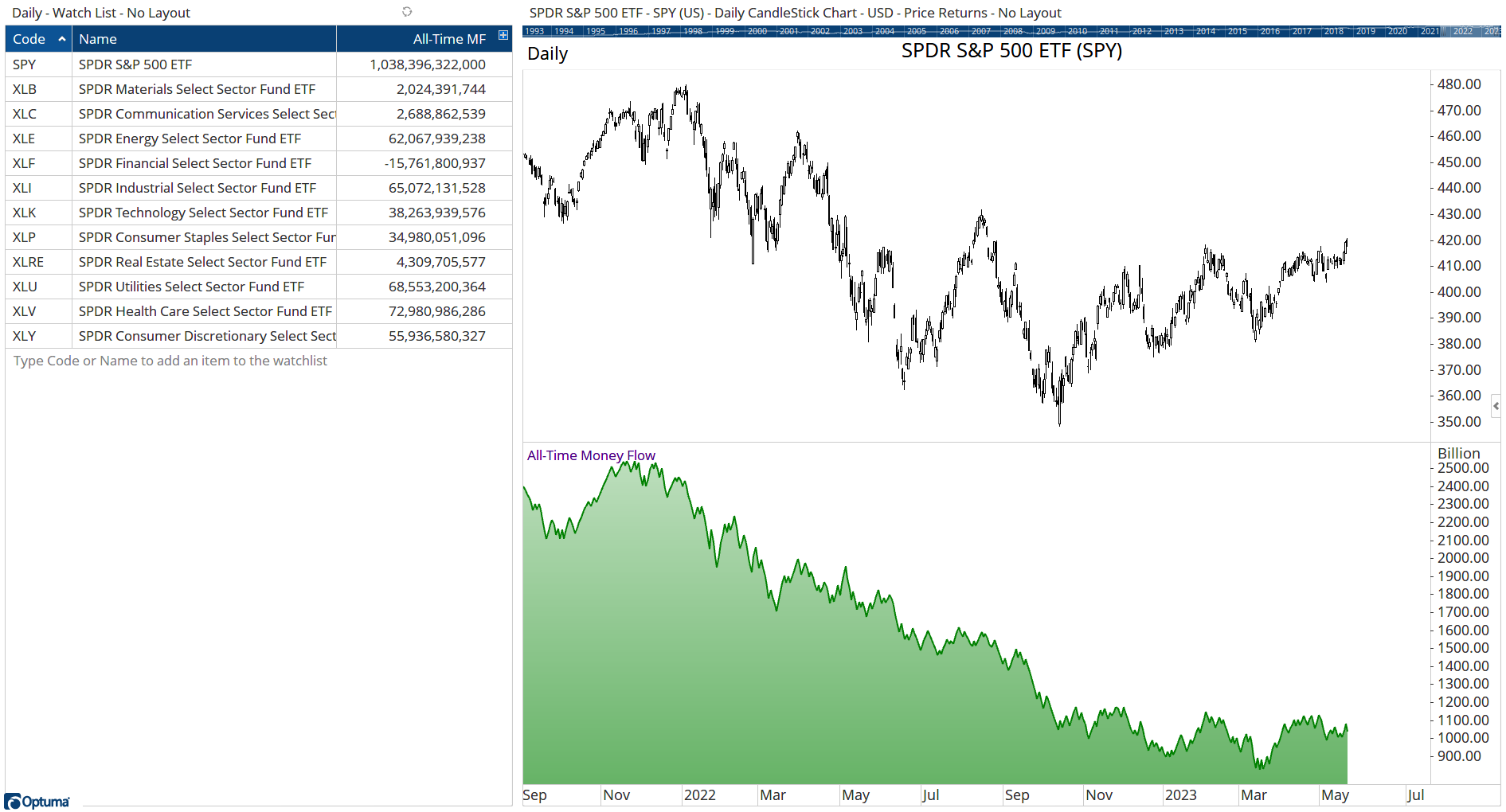Select Page

# Money Flow Script

Optuma Forums Optuma Scripting Money Flow Script

Viewing 3 posts - 1 through 3 (of 3 total)
• Author
Posts
• #70961

Hi,

I am trying to script an indicator that does the following:

• When the security price closes higher, calculate price * volume and add that to the previous value.
• When the security price closes lower, calculate price * volume and subtract that from the previous value.

Not sure what to do to make this work. Here is my attempt:

Thank you for helping.

#70975

Hi Louis,

We have a tool called Turnover with a function TO() which is basically the daily value of trading (close x volume). We can take the TO() value and multiply it by -1 when the IF() condition is false. This will calculate the all-time money flow:#71069Darren